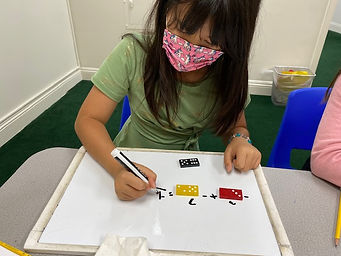Ms. Dawn

Target 1​

Lesson Type:

Continuation

Number Operation

:

Computation

Multiply within 1,000.

1:

Understand there are many ways to multiply multi-digit numbers.

2:

Understand the role of place-value in multiplication strategies.

3:

Use the standard algorithm for solving multi-digit multiplication equations.

4th

Vocabulary:

Partial products, Lattice method, Box method, Standard algorithm, Multi-digit, Place-value, Product

Activities:

1. Students were given a variety of multiplication equations to solve. They could show their thinking by using partial products, Lattice method, Box method, or standard algorithm.

2. Students used partial products to show their understanding of place value multiplication and then needed to explain what they did and why. Also, they needed to tell me what each number represented in place value terms.Home Exploration

Guiding Questions:Absent Students:

Target 2

:

1:

Understand the relationship between positive and negative numbers.

2:

Understand that integers get smaller in value as they move left in order, and larger as they move right in order.

4th

Vocabulary:

Quantities, Positive, Negative, Number line, Value, Integers

Activities:

1. Students solved equations that included positive and negative numbers. They used a number line to show their thinking.

2. Students chose two different dominoes each time and wrote an equation using positive and negative numbers. After writing their equation, students needed to solve it using their knowledge of adding and subtracting positive and negative numbers. Students were allowed to draw number lines as assistance.

3. Students were given a variety of equations adding and subtracting positive and negative numbers. They were able to use the number line to show their understanding. If they didn't use the number line, they needed to explain their thinking to me after solving it.Home Exploration

Guiding Questions:Target 3

:

1:

Identify the correct arithmetic processes based on the information presented in word problems.

2:

Find the key words in word problems that indicate the correct arithmetic process to use: Addition: add, altogether, both, combined, in all, plus, sum, total; Subtraction: difference, fewer, how many/much more, left, less, minus, remains; Multiplication: times, product, each, twice; and Division: how many in each, divided by, quotient, goes into, split evenly.

3:

Find and use the needed information in a word problem to solve.

4th

Vocabulary:

Sum, Product, Difference, Descend, Ascend, Loss, Gain, Below, Above, CUBES

Activities:

1. Students were given a variety of word problems to solve. These word problems dealt with positive and negative numbers. Students drew pictures to help solve the problem.

2. Students were also introduced to the acronym "CUBES." They used this to solve each word problem.

3. Students learned vocabulary words that relates to positive and negative numbers (ex. Descend, Ascend, Loss, Gain, Below, Above, etc).Home Exploration